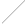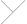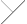Blog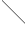# Building Pipelines with Lambdas

The village of Den Haag
Oct. 6, 2018

Consider a toy pipeline:

An off-topic remark is in order now. If you insist on doing everything from scratch, stock up on free time first. Some past Sunday I did not feel like doing much, so I thought I would spend it to write this blog post. I ended up spending it starting to write this blog post. That is, I got stuck with the above diagram. Coding SVG by hand is tedious, so instead I chose to code some Lisp functions that would make it easier to draft flowcharts. As it stands, I am using LaTeX syntax and a custom parser for my blog, but my parser did not support named arguments, only positional ones, and I thought named arguments would be clearer for the flowchart commands, so I had to implement those first. And so it went...

But let us go back to the diagram. I want to implement this pipeline using functional programming where each process is a separate function agnostic of other functions. There is a number of conceptually different approaches to achieve such modularity, I want to try some of them out, and I also want to do a few simple benchmarks. My goal is to organize my understanding of these ideas, but any chance visitor is welcome to tread along.

A good place to start, for comparison purposes, is with a more imperative implementation. (All the code examples are in Lisp, some benchmarks are also for Scheme, Python, and Julia.)

(defun pipeline () (let (buffer) (do ((k 1 (1+ k))) (nil) (let ((j (/ (* (1+ k) k) 2))) (if buffer (let* ((i (pop buffer)) (r (/ (* i j) (do ((x (max i j) y) (y (min i j) (mod x y))) ((= 0 y) x))))) (dolist (d (do ((x r (floor x 10)) (digits nil (cons (mod x 10) digits))) ((= 0 x) digits))) (print d))) (push j buffer))) (finish-output) (sleep 1)))) (pipeline)

This snippet nicely surmises why modularity is important: without it the code become one big mess, or in this case one small mess, very quickly. However, this code has one advantage: it works in an “online” mode. The data is processed the moment it arrives. This feature is important for network applications, for responsive UIs, for processing big data, for parallelism. And we want to preserve this feature when translating the code into a more functional style.

## Callbacks

One way to write a functional program is to let the processes earlier on in the pipeline call the process later on in the pipeline. Further, for prototyping and for modularity purposes it is convenient if the functions are agnostic of one another, and so can be combined into arbitrary pipelines (just like with Unix pipes). This level of modularity can be achieved with callbacks. (For the purpose of testing continuations, which I do later on, I have also rewritten some loops as recursive functions.)

(defun source (dst) (do ((k 1 (1+ k))) (nil) (funcall dst (/ (* (1+ k) k) 2)) (sleep 1))) (defun new-make-pairs (dst) (let (buffer) (lambda (chunk) (if buffer (funcall dst (cons (pop buffer) chunk)) (push chunk buffer))))) (defun split (chunk dst1 dst2) (funcall dst1 chunk) (funcall dst2 chunk)) (defun multiply (chunk dst) (destructuring-bind (x . y) chunk (funcall dst (* x y)))) (defun toy-gcd (chunk dst) (destructuring-bind (x . y) chunk (if (< x y) (toy-gcd (cons y x) dst) (if (= 0 y) (funcall dst x) (toy-gcd (cons y (mod x y)) dst))))) (defun new-combine (dst) (let (buffer) (lambda (chunk) (if buffer (funcall dst (cons (pop buffer) chunk)) (push chunk buffer))))) (defun divide (chunk dst) (destructuring-bind (x . y) chunk (funcall dst (/ x y)))) (defun get-digits (chunk dst) (unless (= 0 chunk) (get-digits (floor chunk 10) dst) (funcall dst (mod chunk 10)))) (defun sink (chunk) (print chunk) (finish-output)) (defun pipeline () (let* ((get-digits (lambda (c) (get-digits c #'sink))) (divide (lambda (c) (divide c get-digits))) (combine (new-combine divide)) (toy-gcd (lambda (c) (toy-gcd c combine))) (multiply (lambda (c) (multiply c combine))) (split (lambda (c) (split c multiply toy-gcd))) (make-pairs (new-make-pairs split))) (source make-pairs))) (pipeline)

The code is longer now, but each separate function is easy to understand, easy to modify, and easy to reuse. There is a cost of wrapping your head around the concept of nested lambdas, but one would hope that to be a one time investment. The new definition for pipeline shows that having Lisp-2 instead of Lips-1 is not always bad.

One subtle point is worth mentioning. If a pipeline is organized with callbacks, like we have it now, then resources can be protected with unwind-protect. No reliance on garbage collection and finilization is necessary. Here is an example.

(defun source (dst) (write-line "Resource opened") (unwind-protect (do ((k 1 (1+ k))) (nil) (funcall dst (/ (* (1+ k) k) 2)) (sleep 1)) (write-line "Resource closed"))) (defun pipeline (size) (prog ((counter 0)) (let* ((sink (lambda (c) (if (<= (incf counter) size) (sink c) (return)))) (get-digits (lambda (c) (get-digits c sink))) (divide (lambda (c) (divide c get-digits))) (combine (new-combine divide)) (toy-gcd (lambda (c) (toy-gcd c combine))) (multiply (lambda (c) (multiply c combine))) (split (lambda (c) (split c multiply toy-gcd))) (make-pairs (new-make-pairs split))) (source make-pairs)))) (pipeline 5)

## Generators

Another way to program the pipeline, which is the opposite of the callback approach, is to let the functions later on in the pipeline request the next value from the functions earlier on in the pipeline. This way would be natural in Python, where there is native support for generators. Lisp does not have generators but they can be implemented in a general way with continuation-passing style. To start off with CPS, let us consider an even simpler pipeline.

With direct style, we have:

(defun yield (v) (print v)) (defun source (start end) (do ((i start (1+ i))) ((= i end)) (yield i))) (source 0 5)

First, let us rewrite the loop as a recursive call:

(defun source (i end) (when (< i end) (yield i) (source (1+ i) end))) (source 0 5)

We can now convert source to CPS now by “inverting” it:

(defun <& (x y k) (if (< x y) (funcall k))) (defun 1+& (x k) (funcall k (1+ x))) (defun yield& (v k) (print v) (funcall k)) (defun source& (i end k) (<& i end (lambda () (yield& i (lambda () (1+& i (lambda (v) (source& v end k)))))))) (source& 0 5 nil)

So far, so good: everything is still working! At this point we already can implement a generator, but I want to use the cl-cont library and its call/cc function, and to go in that direction let us first inline the yield& function.

(defun source& (i end k) (<& i end (lambda () ((lambda (cc) (print i) (funcall cc)) (lambda () (1+& i (lambda (v) (source& v end k)))))))) (source& 0 5 nil)

Up until now we simply have been calling the function print down the pipeline. Doing so is no different from using callbacks. However, this time around we have a continuation, which we can save in the global variable, release control, and then regain control by invoking the continuation.

(defun source& (i end k) (<& i end (lambda () ((lambda (cc) (setq *cont* cc) i) (lambda () (1+& i (lambda (v) (source& v end k)))))))) (defparameter *cont* (lambda () (source& 0 5 nil))) (dotimes (i 6) (print (funcall *cont*)))

Voilà, we have a working generator. Now, instead of rewriting functions into CPS by hand, we can use the cl-cont library. (For those familiar with Scheme, call/cc as implemented by cl-cont is not a true call with continuation. In Scheme, if continuation k is called from some execution branch, the execution will never implicitly return to that branch. In Lisp, the execution will implicitly return to that branch at the end of the with-call/cc block.)

(require "cl-cont") (use-package :cl-cont) (defun/cc source (start end) (do ((i start (1+ i))) ((= i end)) (call/cc (lambda (cc) (setq *cont* cc) i)))) (defparameter *cont* (lambda () (source 0 5))) (dotimes (i 6) (print (funcall *cont*)))

The continuation can be saved in a closure instead of a global variable:

(defun source (start end) (let (cont) (with-call/cc (flet ((source () (do ((i start (1+ i))) ((= i end)) (let/cc cc (setq cont cc) i)))) (setq cont (lambda () (source))))) (lambda () (funcall cont)))) (let ((stream (source 0 5))) (dotimes (i 6) (print (funcall stream))))

We further need a way to stop the iteration once the stream finishes. This can be accomplished, for example, with conditions (exceptions).

(define-condition stop-iteration (error) ()) (defun source (start end) (let (cont) (with-call/cc (flet ((source () (do ((i start (1+ i))) ((= i end)) (let/cc cc (setq cont cc) i)) (error 'stop-iteration))) (setq cont (lambda () (source))))) (lambda () (funcall cont)))) (let ((stream (source 0 5))) (handler-case (loop for i = (funcall stream) do (print i)) (stop-iteration ())))

Finally, we can wrap our new solution in a macro, so that we have a clean syntax for defining and using generators (these particular macros are not fully general, but they will suffice for our purposes).

(require "alexandria") (use-package :alexandria) (defmacro defstream (name args &body body) (with-gensyms (cont cc) `(defun ,name ,args (macrolet ((yield (var) `(let/cc ,',cc (setq ,',cont ,',cc) ,var))) (let (,cont) (with-call/cc (flet ((,name () ,@body (error 'stop-iteration))) (setq ,cont (lambda () (,name))))) (lambda () (funcall ,cont))))))) (defmacro dostream ((var stream) &body body) (with-gensyms (cont start) `(let ((,cont ,stream) (,var nil)) (handler-case (block nil (tagbody ,start (setq ,var (funcall ,cont)) ,@body (go ,start))) (stop-iteration ()))))) (defstream source (start end) (do ((i start (1+ i))) ((= i end)) (yield i))) (dostream (i (source 0 5)) (print i))

The resulting defstream and dostream are as concise as Python code. Kudos to Lisp for making it possible to implement a non-trivial foreign paradigm, and yet make it fill like an existing part of the language. There will be performance costs, of course, and we shall see just how large those are later on. But also, kudos to Python for making generators easy in Python in the first place. In comparison, Julia implements more general tasks, but that makes the syntax for simple generators more cumbersome.

We can implement the pipeline using generators now.

(defstream source () (do ((k 1 (1+ k))) (nil) (yield (/ (* (1+ k) k) 2)) (sleep 1))) (defstream make-pairs (src) (let (buffer) (dostream (chunk src) (if buffer (yield (cons (pop buffer) chunk)) (push chunk buffer))))) (defstream split (src) (dostream (chunk src) (yield chunk) (yield chunk))) (defstream multiply (src) (dostream (chunk src) (destructuring-bind (x . y) chunk (yield (* x y))))) (defstream toy-gcd (src) (labels ((helper (x y) (if (< x y) (helper y x) (if (= 0 y) (yield x) (helper y (mod x y)))))) (dostream (chunk src) (destructuring-bind (x . y) chunk (helper x y))))) (defstream combine (src1 src2) (dostream (x src1) (yield (cons x (funcall src2))))) (defstream divide (src) (dostream (chunk src) (destructuring-bind (x . y) chunk (yield (/ x y))))) (defstream get-digits (src) (labels ((helper (x) (unless (= 0 x) (helper (floor x 10)) (yield (mod x 10))))) (dostream (x src) (helper x)))) (defun sink (src) (dostream (chunk src) (print chunk) (finish-output))) (defun pipeline () (let ((split (split (make-pairs (source))))) (sink (get-digits (divide (combine (multiply split) (toy-gcd split))))))) (pipeline)

With callbacks, each function was written as if processing a single chunk of data. With generators, each function is written as if iterating over all chunks of data, because generators allow us to interrupt a function’s execution. The former style is more concise for 1-to-1 pipelines, becomes contrived for more complex flows, and is not possible in some cases. The latter style, in my opinion, is easier to understand for more complex flows. Here is an example where generators will do the trick, but callbacks are not possible:

On the other hand, unwind-protect is not applicable anymore when generators are used, and if a resource at the beginning of a pipeline needs to be managed, then finilization has to be used.

Lastly, Lisp allows for dynamic binding of global variables. With callbacks, the source can control the behaviour of the sink without threading extra arguments through the whole pipeline, which can be useful when writing a printer. With generators, the sink can control the behaviour of the source, which can be useful when writing a parser.

We discussed top-to-bottom and bottom-to-up control flows, and we programmed them with Lisp language primitives. We can also do concurrency by using OS threads (in languages like Erlang and Go, there are concurrency primitives provided by the language itself—green threads). There is Bordeaux library for using threads in Lisp in a portable way, but let me use SBCL extensions directly, because I want to try those.

The threads code is mostly analogous to generators code. It can be made more concise with an extra macro or two, but let me leave it at that. For simplicity, I also do not control for sizes of pipes (doing so in a production code would be a bad idea).

## Benchmarks

Many of us have learned programming not as computer scientists but as a tool for their own domain. My domain is game-theoretical models and econometrics. That is to say, I never need to write time-critical code. Maybe even the opposite, the longer my models take to solve, the more time I have to chat with colleagues over a cup of coffee. Where does my obsession with ill-designed benchmarks come from then? So, benchmarks.

I have run the code snippets 100 times with triangular series of length 100,000, sans (pause 1) and (print ...). I have also benchmarked Python, Julia, and Scheme implementations of the generators approach, because Python and Julia support generators natively, and because Scheme supports call/cc natively. There is also Golang in the mix as a modern champion for green threads. (Importantly, I use unbuffered channels for a fair comparison with generators.) The tests have been run on AMD Ryzon 3600. I have not invoked any compiler optimization, nor have I used type declarations. (In the table below each row links to the respective code.)

Imperative (Lisp)SBCL 2.1.11.9 s
Callbacks (Lisp)SBCL 2.1.12.4 s
Generators (Lisp)SBCL 2.1.121 s
Generators (Scheme)Chez 9.5.45.0 s
Generators (Python)Python 3.9.228 s
Generators (Julia)Julia 1.6.0165 s

So, callbacks impose relatively little extra penalty in Lisp in comparison with a more imperative implementation. Lisp does not have native generators and implementing them, while possible, is expensive. Python is slow, as would be typical for a benchmark heavy on function calling. Generators in Chez Scheme, implemented on top of call/cc, come clearly ahead of other generators. As for Julia, I decided to benchmark it after I got the results for Scheme and Python, hoping maybe Julia beats Python fair and square. Fat disappointment.

Now, threads. I know thread switching is expensive, but I honestly thought they will take the top spot, especially because there are no restrictions on pipe sizes in the Lisp threads code and all the functions can thus run in parallel. Turns out threads are roughly as slow as Python’s generators. Green threads in Go perform just as poorly. Supposedly, Go excels at async IO, which this benchmark most certainly is not. Still, I was curios if green threads in Go could be used as a light-weight concurrency primitive. Chez Scheme is a faster choice.

I’ve posted this benchmark on reddit, and as mfraddit subsequently pointed out, I’ve cheated somewhat when it comes to big integers. The benchmark, as presented, generates big integers for higher values of the triangular series. In the preceding table, Lisp, Scheme, and Python handle big integers correctly but at the additional computational expense, whereas running Julia and Go code results in integer overflow, which gets silently ignored. In the table below, I’ve redone the computations but explicitly using big integers in Julia and Go. While I had no intention benchmarking big integer operations at the start, and this is all rather accidental, the results do seem to suggest that standard big integer libraries in Julia and Go aren’t very good.

Generators (Julia)Julia 1.6.0213 s

On a closing note, my example is a contrived one: most processing time is spend moving data chunks around instead of processing them. If processing time becomes significant, there will be little difference between callbacks and generators, and the threads—green or not—are likely to come on top.

## Postscriptum

The benchmarks were updated on 30 May, 2021. Notably, back in 2018 I used Chicken Scheme and surprisingly it was slower than the non-native generators in Lisp. In 2021 I switched to Chez and Chez is just faster, at least on this benchmark.

Search Algorithms
RePEc Map
RePEc Trends
# Hash Tables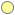Lambda Pipes
On Lisp
Motorbike Diaries
Raspberry Pi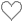2
Search Algorithms
RePEc Map
RePEc Trends
# Hash TablesLambda Pipes
On Lisp
Motorbike Diaries
Raspberry Pi